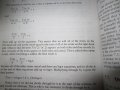# Solving recurrence of Merge Sort

#### zulfi100

Joined Jun 7, 2012
656
Hi,
I cant understand why we get log n while solving recurrence of Merge Sort. I have attached the book content. It replaces the ' 1 ' on RHS by log n. It says" because all of the other terms cancel & there are log n equations and so all the 1s at the end of these equations add up to log n."

If n is 8, then we would split list into 4, 2 and 1. This means that there would be 3 equations, but log 8 is not 3 mathematically.

Can some body please explain me the number of equations for n=8?

Zulfi.#### WBahn

Joined Mar 31, 2012
28,163
The base that applies here is base 2. The base-2 logarithm of 8 is 3.

#### zulfi100

Joined Jun 7, 2012
656
The base that applies here is base 2. The base-2 logarithm of 8 is 3.
Hi,
Thanks for your answer. In my calculator, i have log key (which 10 to the power x written on its top) and ln key (which has e raised to the power x written on its top). How can we calculate base-2 logarithm using calculator?

Zulfi.

#### djsfantasi

Joined Apr 11, 2010
8,783
Divide $$log_{10}(number)$$ by $$log_{10}(2)$$

#### WBahn

Joined Mar 31, 2012
28,163
Hi,
Thanks for your answer. In my calculator, i have log key (which 10 to the power x written on its top) and ln key (which has e raised to the power x written on its top). How can we calculate base-2 logarithm using calculator?

Zulfi.
The best way is by understanding what a logarithm is -- if you do that, then you can quickly derive how to calculate the log to an arbitrary base in just a couple lines of algebra.

A logarithm, by definition, is the exponent that a base must be raised to in order to equal a number. So we know that

$$1000 \; = \; 10^3$$

So, by definition, 3 is the base 10 logarithm of 1000.

If we want the base B logarithm of a some number x, then we are looking for the value y such that

$$x \; = \; B^y$$

So we simply solve this for y by taking the logarithm of both sides:

$$\ln(x) \; = \; y \, \ln(B) \, y \; = \; \frac{\ln(x)}{\ln(B)}$$

Note that it does not matter which base you take the logarithm with respect to -- as long as you use the same base for both operations.

So for the base-2 logarithm, you simply have

$$y \; = \; \frac{\ln(x)}{\ln(2)} \; = \; \frac{\log_{10}(x)}{\log_{10}(2)}$$